Updating search results...

# 72 Results

View
Selected filters:
• cluster-7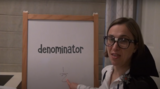Unrestricted Use
CC BY
Rating
0.0 stars

This lesson is Day 10 in a series of 12 lessons around fraction equivalences and comparisons.

This video lesson serves as a review of equpartitioning in order to determine the fractional part of an area or length. This is a necessary foundational skill for comparing and finding equivalent fractions.

Subject:
Mathematics
Material Type:
Activity/Lab
Interactive
Lesson
Simulation
Author:
Dawne Coker
06/25/2020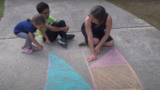Unrestricted Use
CC BY
Rating
0.0 stars

This lesson is Day 11 in a series of 12 lessons around fraction equivalences and comparisons.

This video lesson reviews parts of a whole, comparing fractions, and equivalent fractions. No materials are needed.

Subject:
Mathematics
Material Type:
Activity/Lab
Interactive
Lesson
Simulation
Author:
Dawne Coker
06/25/2020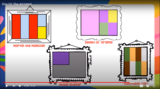Unrestricted Use
CC BY
Rating
0.0 stars

This lesson is Day 12 in a series of 12 lessons around fraction equivalences and comparisons.

Use your math skills to help detectives solve the crime. This video lesson reviews fractions of an area, fractions on a number line, and equivalent fractions. No materials are required.

Subject:
Mathematics
Material Type:
Activity/Lab
Interactive
Lesson
Simulation
Author:
Dawne Coker
06/25/2020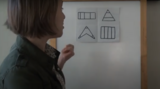Unrestricted Use
Public Domain
Rating
0.0 stars

This lesson is Day 1 in a series of 12 lessons around fraction equivalences and comparisons.

This video lesson encourages students to reason about and visualize fractions. The lesson focuses on the ideas that fractions are fair shares and that fractions can be compared. This lesson assumes that students have some initial experiences with fractions.

Subject:
Mathematics
Material Type:
Interactive
Lesson
Presentation
Simulation
Author:
Dawne Coker
06/25/2020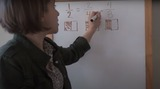Unrestricted Use
Public Domain
Rating
0.0 stars

This lesson is Day 2 in a series of 12 lessons around fraction equivalences and comparisons.

This video lesson uses paper folding to introduce the concept of equivalent fractions. To complete this lesson, make sure you have a square piece of paper (any size) and a crayon

Subject:
Mathematics
Material Type:
Activity/Lab
Interactive
Lesson
Simulation
Author:
Dawne Coker
06/25/2020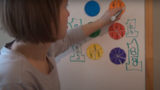Unrestricted Use
CC BY
Rating
0.0 stars

This lesson is Day 2 in a series of 12 lessons around fraction equivalences and comparisons.

This video lesson introduces the idea that fractions can be used to represent 1 whole. To engage in this lesson, students may use virtual circle models from Toy Theater (https://toytheater.com/fraction-circles/)

Subject:
Mathematics
Material Type:
Activity/Lab
Interactive
Lesson
Simulation
Author:
Dawne Coker
06/25/2020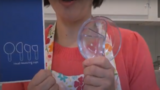Unrestricted Use
CC BY
Rating
0.0 stars

This lesson is Day 4 in a series of 12 lessons around fraction equivalences and comparisons.

This video lesson looks at how we use fractions in the kitchen. Students are challenged to use circle models to find equivalent fractions for a recipe. Students may use virtual circe models from the Toy Theater (https://toytheater.com/fraction-circles/).

Subject:
Mathematics
Material Type:
Activity/Lab
Interactive
Lesson
Simulation
Author:
Dawne Coker
06/25/2020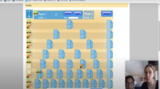Unrestricted Use
CC BY
Rating
0.0 stars

This lesson is Day 2 in a series of 12 lessons around fraction equivalences and comparisons.

This game may be played with one or two people. It reviews fractions on a number line, adding fraction with like denominators, and equivalent fractions. For an extra challenge, you can even break up your moves across multiple tracks (e.g., if your card reads 1/2, you could move 1/4 and 2/8).

https://www.nctm.org/Classroom-Resources/Illuminations/Interactives/Playing-Fraction-Track/

Subject:
Mathematics
Material Type:
Activity/Lab
Interactive
Lesson
Simulation
Author:
Dawne Coker
06/25/2020Unrestricted Use
CC BY
Rating
0.0 stars

This lesson is Day 6 in a series of 12 lessons around fraction equivalences and comparisons.

This video lesson teaches students how to create bar models to compare lengths/distances. Students will need a piece of paper, ruler, scissors, and a pencil. These bar models will also be used for Day 7's lesson.

Subject:
Mathematics
Material Type:
Activity/Lab
Interactive
Lesson
Simulation
Author:
Dawne Coker
06/25/2020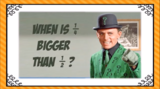Unrestricted Use
CC BY
Rating
0.0 stars

This video lesson introduces the idea that when comparing two fractions, they must refer to the same size whole. Then, students use fraction strips to compare fractions. Students may use virtual fraction strips from Toy Theater (https://toytheater.com/fraction-strips/)

Subject:
Mathematics
Material Type:
Activity/Lab
Interactive
Lesson
Simulation
Author:
Dawne Coker
06/25/2020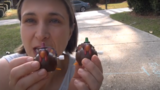Unrestricted Use
CC BY
Rating
0.0 stars

This lesson is Day 8 in a series of 12 lessons around fraction equivalences and comparisons.

This video lesson introduces the number as a tool for representing and comparing fractions. Students will need a black piece of a paper and a pencil with a good eraser.

Subject:
Mathematics
Material Type:
Activity/Lab
Interactive
Lesson
Simulation
Author:
Dawne Coker
06/25/2020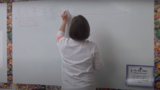Unrestricted Use
CC BY
Rating
0.0 stars

This lesson is Day 9 in a series of 12 lessons around fraction equivalences and comparisons.

This video lesson introduces students to fractions beyond 1 using a choral counting routine. Students connect the fractions to points on a number line and bar models. For this lesson, students need a piece of blank paper and a pencil.

Subject:
Mathematics
Material Type:
Activity/Lab
Interactive
Lesson
Simulation
Author:
Dawne Coker
06/25/2020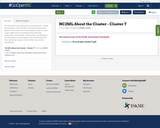Only Sharing Permitted
CC BY-NC-ND
Rating
0.0 stars

This document is the About the Cluster document created by the authors of the NC2ML Instructional Frameworks. Read this document prior to teaching the cluster in order to get insight into the clustering of the standards, mathematics to be taught, and important considerations.

This document is not remixable since the document has been written by creators of the NC2ML Instructional Frameworks.

Subject:
Mathematics
Material Type:
Reference Material
11/11/2019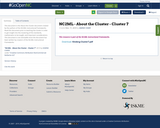Only Sharing Permitted
CC BY-NC-ND
Rating
0.0 stars

This document is the About the Cluster document created by the authors of the NC2ML Instructional Frameworks. Read this document prior to teaching the cluster in order to get insight into the clustering of the standards, mathematics to be taught, and important considerations.

This document is not remixable since the document has been written by creators of the NC2ML Instructional Frameworks.

Subject:
Mathematics
Material Type:
Reference Material
11/11/2019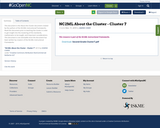Only Sharing Permitted
CC BY-NC-ND
Rating
0.0 stars

This document is the About the Cluster document created by the authors of the NC2ML Instructional Frameworks. Read this document prior to teaching the cluster in order to get insight into the clustering of the standards, mathematics to be taught, and important considerations.

This document is not remixable since the document has been written by creators of the NC2ML Instructional Frameworks.

Subject:
Mathematics
Material Type:
Reference Material
11/11/2019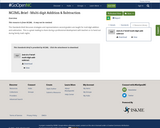Unrestricted Use
CC BY
Rating
0.0 stars

This resource is from NC2ML.&nbsp; It may not be remixed.This Standards Brief discusses strategies and representations second graders are taught for mult-digit addition and subtraction.&nbsp; This is a great reading to share during a professional development with teachers or to hand out during family math nights.&nbsp;&nbsp;

Subject:
Mathematics
Material Type:
Diagram/Illustration
Reference Material
Teaching/Learning Strategy
Author:
DAWNE COKER
06/20/2020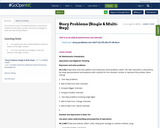Conditional Remix & Share Permitted
CC BY-NC-SA
Rating
0.0 stars

This resource is part of Tools4NCTeachers.

In this lesson students use the open number and various strategies to solve two-step story problems.

Subject:
Mathematics
Material Type:
Lesson
11/06/2019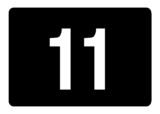Unrestricted Use
CC BY
Rating
0.0 stars

This resource is from Tools4NCTeachers.&nbsp;&nbsp;In this lesson, students will be figuring out the addends that add up to the correct sums.&nbsp; They will also be creating and solving equations with more than two addends.Extension and differentiation ideas are provided.

Subject:
Mathematics
Material Type:
Activity/Lab
Lesson
Lesson Plan
Author:
DAWNE COKER
06/23/2020Unrestricted Use
CC BY
Rating
0.0 stars

This resource is from Tools4NCTeachers.&nbsp;&nbsp;This is a set of five tasks, which may be&nbsp;used for instruction or assessment.&nbsp; Each file contains a separate task and rubric.&nbsp;&nbsp;Remix this resource to include extension ideas or addtional tasks.

Subject:
Mathematics
Material Type:
Activity/Lab
Assessment
Formative Assessment
Author:
DAWNE COKER
06/08/2020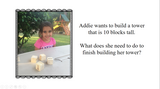Unrestricted Use
CC BY
Rating
0.0 stars

This resource is from Tools4NCTeachers.The goals of this lesson are to a) use objects or drawings to find the number that makes 10 when added to a known number, and b) record answers using drawings or expressions.Remix this lesson to share addtional problems, extension ideas, or student work samples.

Subject:
Mathematics
Material Type:
Activity/Lab
Lesson
Lesson Plan
Author:
DAWNE COKER